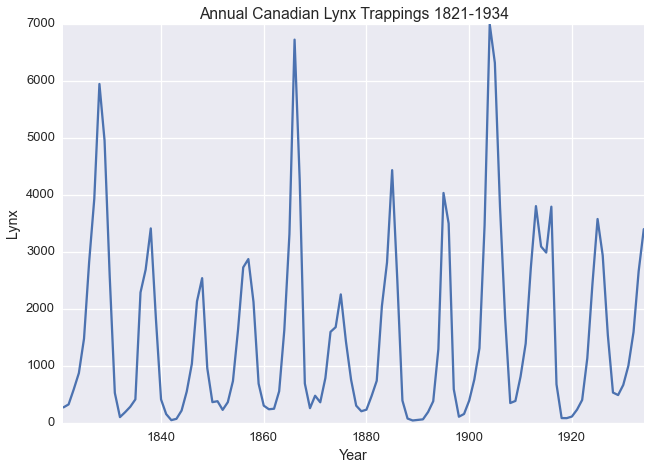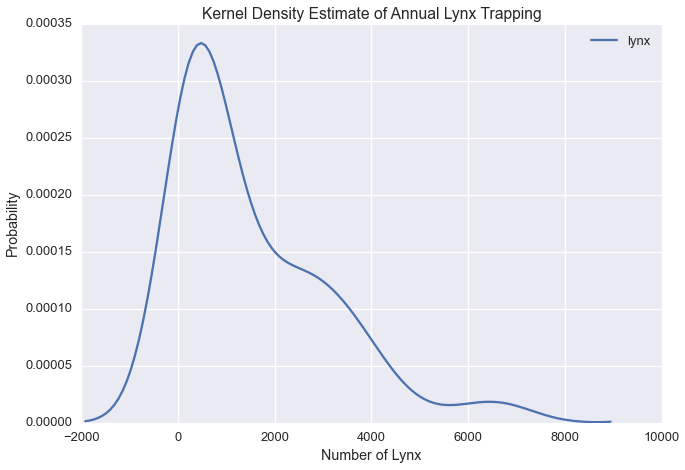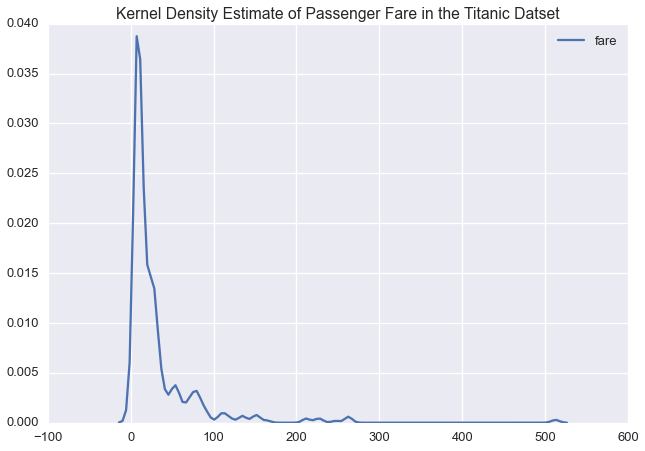# Univariate Data with the Normal Inverse Chi-Square Distribution¶

One of the simplest examples of data is univariate data

Let’s consider a timeseries example:

The Annual Canadian Lynx Trappings Dataset as described by Campbel and Walker 1977 contains the number of Lynx trapped near the McKenzie River in the Northwest Territories in Canada between 1821 and 1934.

import pandas as pd
import numpy as np
import seaborn as sns
import matplotlib.pyplot as plt
%matplotlib inline
sns.set_context('talk')
sns.set_style('darkgrid')

lynx = pd.read_csv('https://vincentarelbundock.github.io/Rdatasets/csv/datasets/lynx.csv',
index_col=0)

lynx = lynx.set_index('time')

lynx
time
1821 269
1822 321
1823 585
1824 871
1825 1475
lynx.plot(legend=False)
plt.xlabel('Year')
plt.ylabel('Lynx')

<matplotlib.text.Text at 0x1129d0090>Let’s plot the kernel density estimate of annual lynx trapping

sns.kdeplot(lynx['lynx'])
plt.title('Kernel Density Estimate of Annual Lynx Trapping')
plt.ylabel('Probability')
plt.xlabel('Number of Lynx')

<matplotlib.text.Text at 0x1129d0410>Our plot suggests there could be three modes in the Lynx data.

In modeling this timeseries, we could assume that the number of lynx trapped in a given year is falls into one of $$k$$ states, which are normally distributed with some unknown mean $$\mu_i$$ and variance $$\sigma^2_i$$ for each state

In the case of our Lynx data

$\forall i \in [1,...,k] \hspace{2mm} p(\text{lynx trapped}| \text{state} = i) \sim \mathcal{N}(\mu_i, \sigma^2_i)$

Now let’s consider demographics data from the Titanic Dataset

The Titanic Dataset contains information about passengers of the Titanic.

ti = sns.load_dataset('titanic')

survived pclass sex age sibsp parch fare embarked class who adult_male deck embark_town alive alone
0 0 3 male 22 1 0 7.2500 S Third man True NaN Southampton no False
1 1 1 female 38 1 0 71.2833 C First woman False C Cherbourg yes False
2 1 3 female 26 0 0 7.9250 S Third woman False NaN Southampton yes True
3 1 1 female 35 1 0 53.1000 S First woman False C Southampton yes False
4 0 3 male 35 0 0 8.0500 S Third man True NaN Southampton no True

Passenger age and fare are both real valued. Are they related? Let’s examine the correlation matrix

ti[['age','fare']].dropna().corr()

age fare
age 1.000000 0.096067
fare 0.096067 1.000000

Since the correlation is between the two variables is zero, we can model these two real valued columns independently.

Let’s plot the kernel density estimate of each variable

sns.kdeplot(ti['age'])
plt.title('Kernel Density Estimate of Passenger Age in the Titanic Datset')

<matplotlib.text.Text at 0x117426c50>sns.kdeplot(ti['fare'])
plt.title('Kernel Density Estimate of Passenger Fare in the Titanic Datset')

<matplotlib.text.Text at 0x117475310>Given the long tail in the fare price, we might want to model this variable on a log scale:

ti['logfare'] = np.log(ti['fare'])
ti[['age','logfare']].dropna().corr()

age logfare
age 1.000000 0.135352
logfare 0.135352 1.000000

Again, logfare and age have near zero correlation, so we can again model these two variables independently

Let’s see what a kernel density estimate of log fare would look like

sns.kdeplot(ti['logfare'])
plt.title('Kernel Density Estimate of Log Passenger Fare in the Titanic Datset')

<matplotlib.text.Text at 0x1175a5110>In logspace, passenger fare is multimodal, suggesting that we could model this variable with a normal distirbution

If we were to model the passenger list using our Mixture Model, we would have separate likelihoods for logfare and age

$\forall i \in [1,...,k] \hspace{2mm} p(\text{logfare}|\text{cluster}=i)=\mathcal{N}(\mu_{i,l}, \sigma^2_{i,l})$
$\forall i \in [1,...,k] \hspace{2mm} p(\text{age}|\text{cluster}=c)=\mathcal{N}(\mu_{i,a}, \sigma^2_{i,a})$

Often, real value data is assumed to be normally distributed.

To learn the latent variables, $$\mu_i$$ $$\sigma^2_i$$, we would use a normal inverse-chi-square likelihood

The normal inverse-chi-square likelihood is the conjugate univariate normal likelihood in data microscopes. We also have normal likelihood, the normal inverse-wishart likelihood, optimized for multivariate datasets.

It is important to model univariate normal data with this likelihood as it acheives superior performance on univariate data.

In both these examples, we found variables that were amenable to being modeled as univariate normal:

1. Univariate datasets
2. Datasets containing real valued variables with near zero correlation

To import our univariate normal inverse-chi-squared likelihood, call:

from microscopes.models import nich as normal_inverse_chisquared